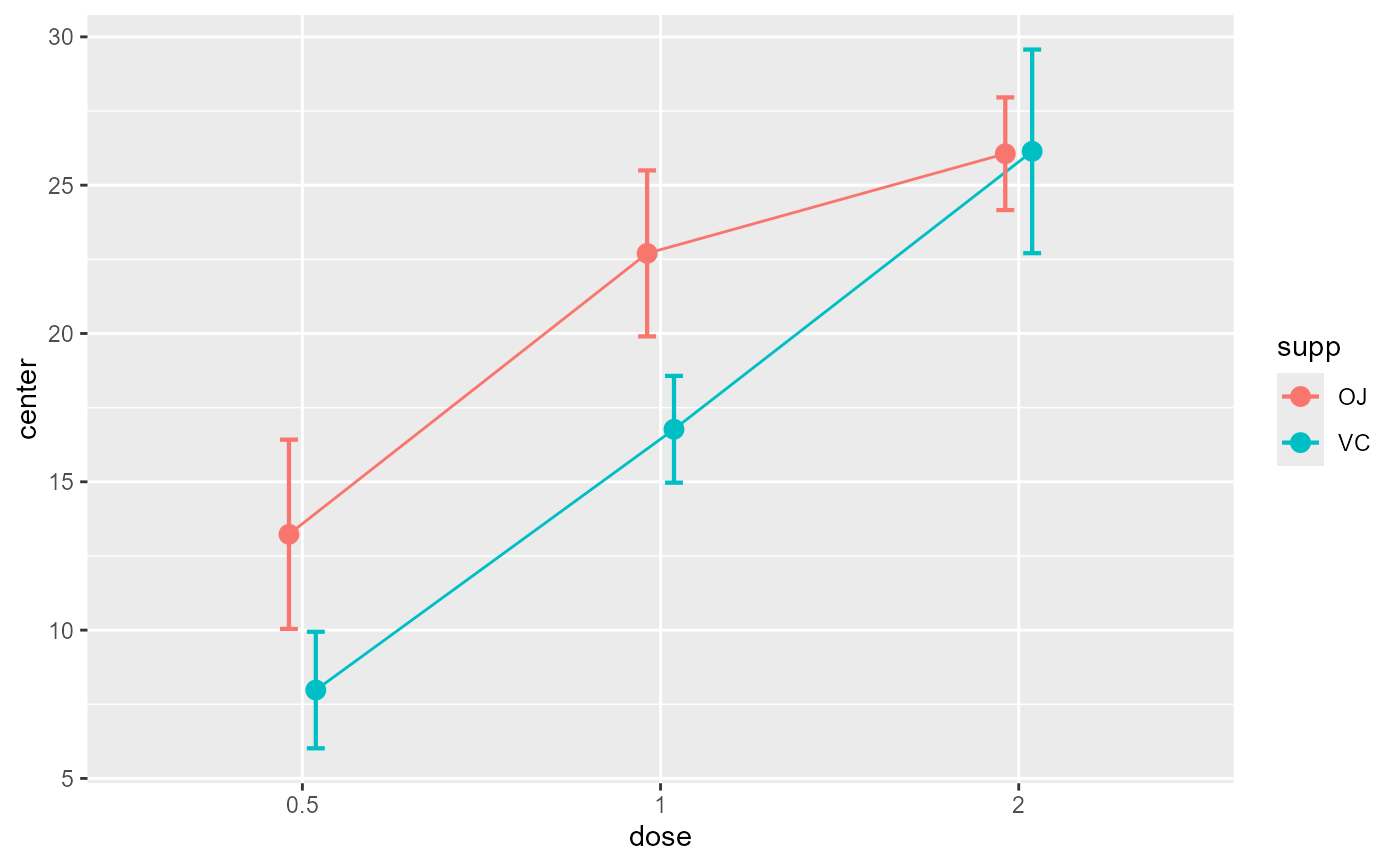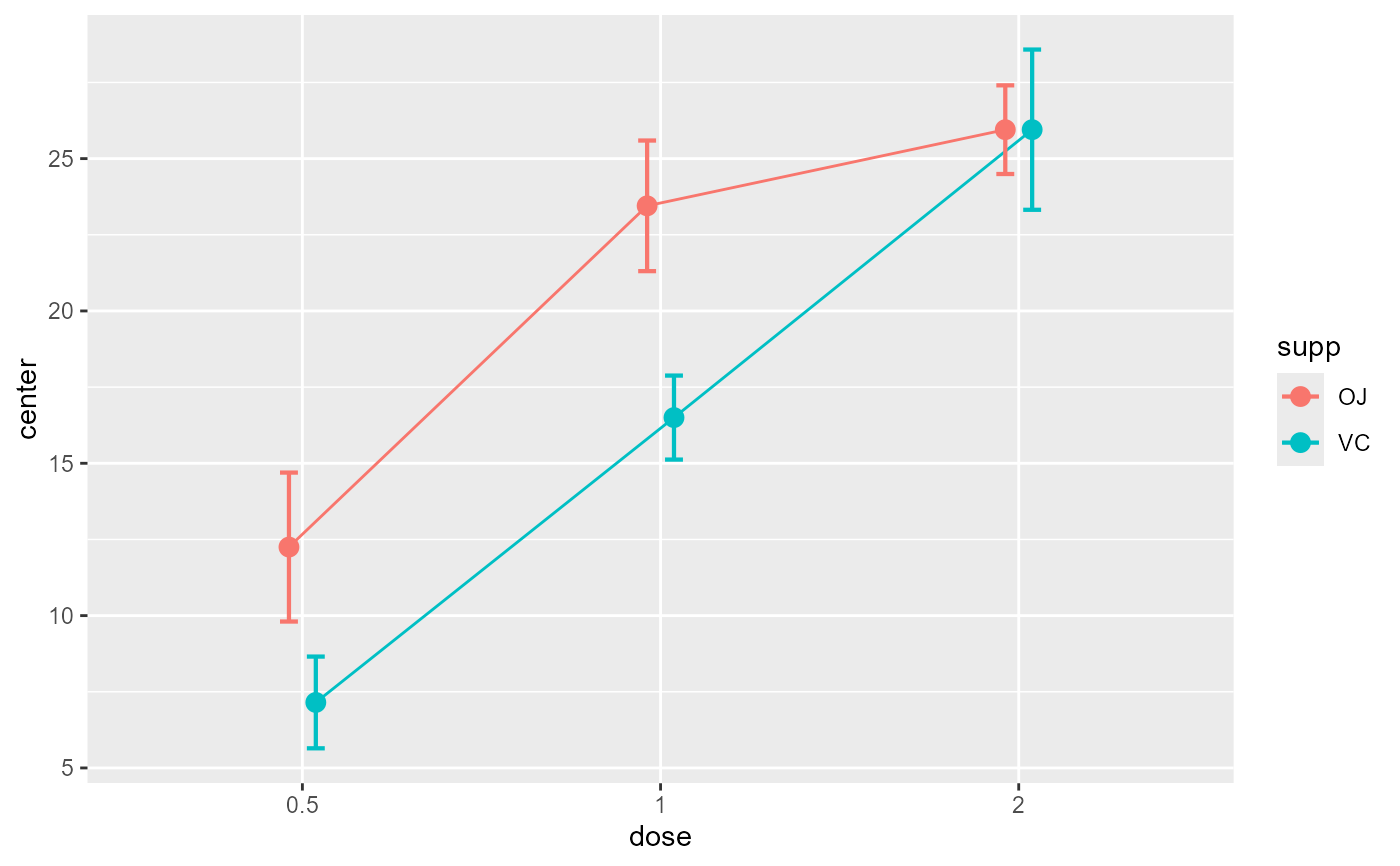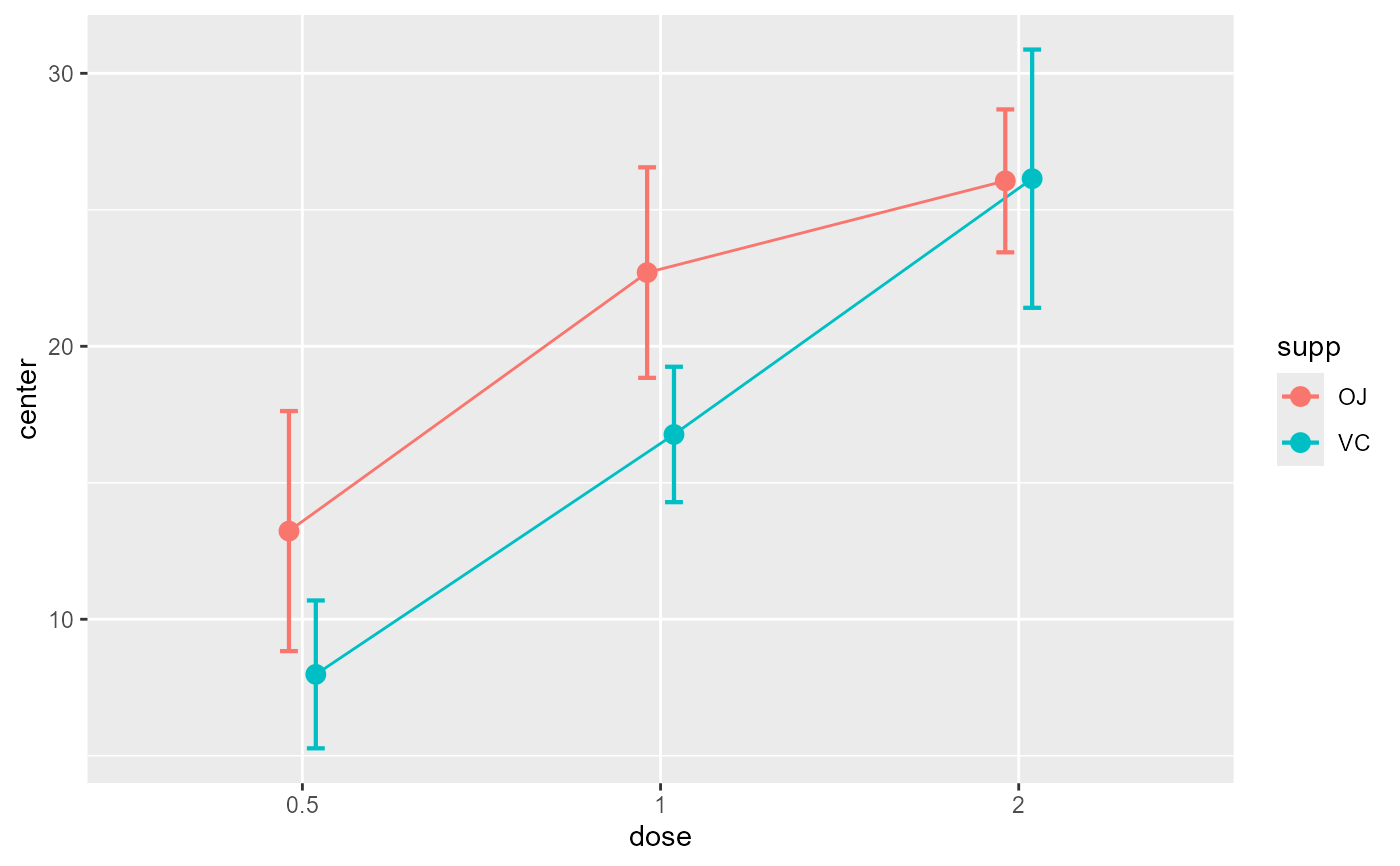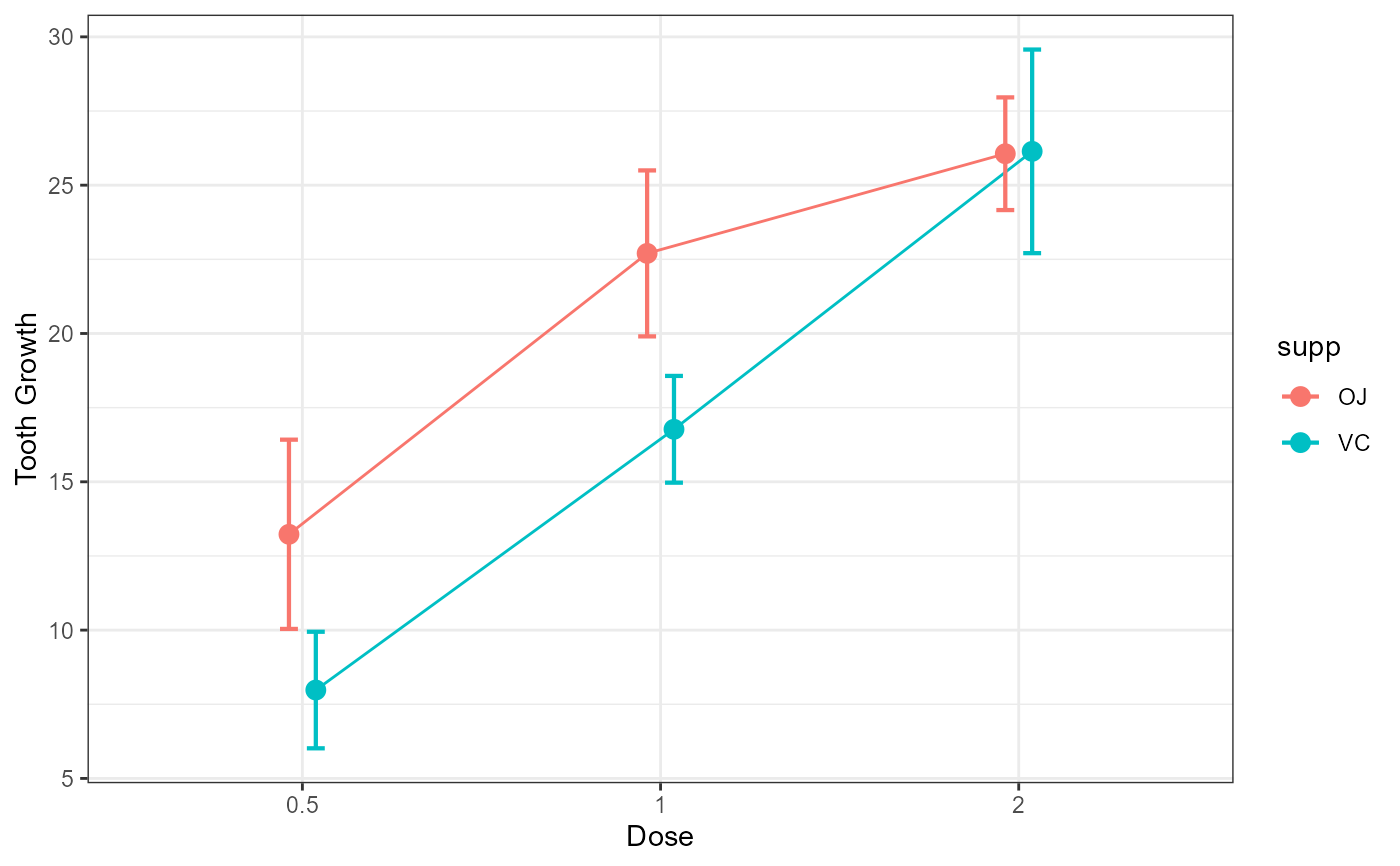The function suberbPlot() plots standard error or confidence interval for various descriptive statistics under various designs, sampling schemes, population size and purposes, according to the suberb framework. See (Cousineau et al. 2021) for more.

superbPlot(
data,
BSFactors = NULL,
WSFactors = NULL,
WSDesign = "fullfactorial",
factorOrder = NULL,
variables,
statistic = "mean",
errorbar = "CI",
gamma = 0.95,
adjustments = list(purpose = "single", popSize = Inf, decorrelation = "none",
samplingDesign = "SRS"),
showPlot = TRUE,
plotStyle = "bar",
preprocessfct = NULL,
postprocessfct = NULL,
clusterColumn = "",
...
)

## Arguments

data Dataframe in wide format The name of the columns containing the between-subject factor(s) The name of the within-subject factor(s) the within-subject design if not a full factorial design (default "fullfactorial") Order of factors as shown in the graph (in that order: x axis, groups, horizontal panels, vertical panels) The dependent variable(s) as strings The summary statistic function to use as a string The function that computes the error bar. Should be "CI" or "SE" or any function name if you defined a custom function. Default to "CI" The coverage factor; necessary when errorbar == "CI". Default is 0.95. List of adjustments as described below. Default is adjustments = list(purpose = "single", popSize = Inf, decorrelation = "none", samplingDesign = "SRS") Defaults to TRUE. Set to FALSE if you want the output to be the summary statistics and intervals. The type of object to plot on the graph. See full list below. Defaults to "bar". is a transform (or vector of) to be performed first on data matrix of each group is a transform (or vector of) used in conjunction with samplingDesign = "CRS", indicates which column contains the cluster membership In addition to the parameters above, superbPlot also accept a number of optional arguments that will be transmitted to the plotting function, such as pointParams (a list of ggplot2 parameters to input inside geoms; see ?geom_bar2) and errorbarParams (a list of ggplot2 parameters for geom_errorbar; see ?geom_errorbar)

## Value

a plot with the correct error bars or a table of those summary statistics. The plot is a ggplot2 object with can be modified with additional declarations.

## Details

The possible adjustements are the following

• popsize: Size of the population under study. Defaults to Inf

• purpose: The purpose of the comparisons. Defaults to "single". Can be "single", "difference", or "tryon".

• decorrelation: Decorrelation method for repeated measure designs. Chooses among the methods "CM", "LM", "CA" or "none". Defaults to "none".

• samplingDesign: Sampling method to obtain the sample. implemented sampling is "SRS" (Simple Randomize Sampling) and "CRS" (Cluster-Randomized Sampling).

In version 0.9.5, the layouts for plots are the following:

• "bar" Shows the summary statistics with bars and error bars;

• "line" Shows the summary statistics with lines connecting the conditions over the first factor;

• "point" Shows the summary statistics with isolated points

• "pointjitter" Shows the summary statistics along with jittered points depicting the raw data;

• "pointjitterviolin" Also adds violin plots to the previous layout

• "pointindividualline" Connects the raw data with line along the first factor (which should be a repeated-measure factor)

• "raincloud" Illustrates the distribution with a cloud (half_violin_plot) and jittered dots next to it. Looks better when coordinates are flipped +coord_flip().

Cousineau D, Goulet M, Harding B (2021). “Summary plots with adjusted error bars: The superb framework with an implementation in R.” Advances in Methods and Practices in Psychological Science, 4, 1--18. doi: 10.1177/25152459211035109 .

## Examples

# Basic example using a built-in dataframe as data.
# By default, the mean is computed and the error bar are 95% confidence intervals
superbPlot(ToothGrowth, BSFactors = c("dose", "supp"),
variables = "len")# Example changing the summary statistics to the median and
# the error bar to 80% confidence intervals
superbPlot(ToothGrowth, BSFactors = c("dose", "supp"),
variables = "len", statistic = "median", errorbar = "CI", gamma = .80)# Example introducing adjustments for pairwise comparisons
# and assuming that the whole population is limited to 200 persons
superbPlot(ToothGrowth, BSFactors = c("dose", "supp"),
variables = "len",
adjustments = list( purpose = "difference", popSize = 200) )# This example adds ggplot directives to the plot produced
library(ggplot2)
superbPlot(ToothGrowth, BSFactors = c("dose", "supp"),
variables = "len") +
xlab("Dose") + ylab("Tooth Growth") +
theme_bw()# This example is based on repeated measures
library(lsr)
library(gridExtra)
options(superb.feedback = 'none') # shut down 'warnings' and 'design' interpretation messages

# define shorter column names...
names(Orange) <- c("Tree","age","circ")
# turn the data into a wide format
Orange.wide <- longToWide(Orange, circ ~ age)

# Makes the plots two different way:
p1=superbPlot( Orange.wide, WSFactors = "age(7)",
variables = c("circ_118","circ_484","circ_664","circ_1004","circ_1231","circ_1372","circ_1582"),
adjustments = list(purpose = "difference", decorrelation = "none")
) +
xlab("Age level") + ylab("Trunk diameter (mm)") +
coord_cartesian( ylim = c(0,250) ) + labs(title="Basic confidence intervals")
p2=superbPlot( Orange.wide, WSFactors = "age(7)",
variables = c("circ_118","circ_484","circ_664","circ_1004","circ_1231","circ_1372","circ_1582"),
adjustments = list(purpose = "difference", decorrelation = "CA")
) +
xlab("Age level") + ylab("Trunk diameter (mm)") +
coord_cartesian( ylim = c(0,250) ) + labs(title="Decorrelated confidence intervals")
grid.arrange(p1,p2,ncol=2)